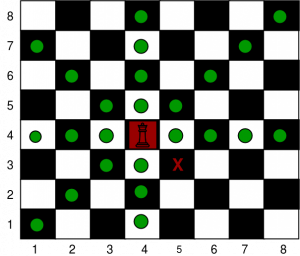GeeksforGeeks App
Open AppBrowser
Continue

# Minimum number of moves required to reach the destination by the king in a chess board

Given four integers sourceX, sourceY, destinationX and destinationY which represent the source and destination coordinates on a chessboard. The task is to find the minimum number of moves required by the king to reach from source to destination.
A king can move to the square that has a common side or a common vertex with the square the king is currently in (generally there are 8 different squares he can move to).Print path using L, R, U, D, LU, LD, RU and RD where L, R, U and D represent left, right, up and down respectively.

Examples:

Input: sourceX = 4, sourceY = 4, destinationX = 3, destinationY = 5
Output:
DR

Input: sourceX = 4, sourceY = 4, destinationX = 7, destinationY = 0
Output:
UL
UL
UL

Approach: Move in the diagonal direction towards the destination until the king reaches same column or same row as the destination, then move towards the destination in a straight line.

Below is the implementation of the above approach:

## C++

 `// C++ program to Find the minimum number of moves required to``// reach the destination by the king in a chess board``#include ``using` `namespace` `std;` `// function to Find the minimum number of moves required to``// reach the destination by the king in a chess board``void` `MinSteps(``int` `SourceX, ``int` `SourceY, ``int` `DestX, ``int` `DestY)``{``    ``// minimum number of steps``    ``cout << max(``abs``(SourceX - DestX), ``abs``(SourceY - DestY)) << endl;` `    ``// while the king is not in the same row or column``    ``// as the destination``    ``while` `((SourceX != DestX) || (SourceY != DestY)) {` `        ``// Go up``        ``if` `(SourceX < DestX) {``            ``cout << ``'U'``;``            ``SourceX++;``        ``}` `        ``// Go down``        ``if` `(SourceX > DestX) {``            ``cout << ``'D'``;``            ``SourceX--;``        ``}` `        ``// Go left``        ``if` `(SourceY > DestY) {``            ``cout << ``'L'``;``            ``SourceY--;``        ``}` `        ``// Go right``        ``if` `(SourceY < DestY) {``            ``cout << ``'R'``;``            ``SourceY++;``        ``}` `        ``cout << endl;``    ``}``}` `// Driver code``int` `main()``{``    ``int` `sourceX = 4, sourceY = 4;``    ``int` `destinationX = 7, destinationY = 0;` `    ``MinSteps(sourceX, sourceY, destinationX, destinationY);` `    ``return` `0;``}`

## Java

 `// Java program to Find the minimum``// number of moves required to reach``// the destination by the king in a``// chess board``import` `java.io.*;` `class` `GFG``{` `// function to Find the minimum number``// of moves required to reach the``// destination by the king in a chess board``static` `void` `MinSteps(``int` `SourceX, ``int` `SourceY,``                     ``int` `DestX, ``int` `DestY)``{``    ``// minimum number of steps``    ``System.out.println(Math.max(Math.abs(SourceX - DestX),``                     ``Math.abs(SourceY - DestY)));` `    ``// while the king is not in the same``    ``// row or column as the destination``    ``while` `((SourceX != DestX) ||``           ``(SourceY != DestY))``    ``{` `        ``// Go up``        ``if` `(SourceX < DestX)``        ``{``            ``System.out.print( ``'U'``);``            ``SourceX++;``        ``}` `        ``// Go down``        ``if` `(SourceX > DestX)``        ``{``            ``System.out.println( ``'D'``);``            ``SourceX--;``        ``}` `        ``// Go left``        ``if` `(SourceY > DestY)``        ``{``            ``System.out.print( ``'L'``);``            ``SourceY--;``        ``}` `        ``// Go right``        ``if` `(SourceY < DestY)``        ``{``            ``System.out.print( ``'R'``);``            ``SourceY++;``        ``}` `        ``System.out.println();``    ``}``}` `// Driver code``public` `static` `void` `main (String[] args)``{``    ``int` `sourceX = ``4``, sourceY = ``4``;``    ``int` `destinationX = ``7``, destinationY = ``0``;` `    ``MinSteps(sourceX, sourceY,``             ``destinationX, destinationY);``}``}` `// This code is contributed by inder_verma`

## Python3

 `# Python 3 program to Find the minimum number of moves required to``# reach the destination by the king in a chess board` `# function to Find the minimum number of moves required to``# reach the destination by the king in a chess board``def` `MinSteps(SourceX, SourceY, DestX, DestY):``    ``# minimum number of steps``    ``print``(``max``(``abs``(SourceX ``-` `DestX), ``abs``(SourceY ``-` `DestY)))` `    ``# while the king is not in the same row or column``    ``# as the destination``    ``while` `((SourceX !``=` `DestX) ``or` `(SourceY !``=` `DestY)):``        ``# Go up``        ``if` `(SourceX < DestX):``            ``print``(``'U'``,end ``=` `"")``            ``SourceX ``+``=` `1``        ` `        ``# Go down``        ``if` `(SourceX > DestX):``            ``print``(``'D'``,end ``=` `"")``            ``SourceX ``-``=` `1``        ` `        ``# Go left``        ``if` `(SourceY > DestY):``            ``print``(``'L'``)``            ``SourceY ``-``=` `1``        ` `        ``# Go right``        ``if` `(SourceY < DestY):``            ``print``(``'R'``,end ``=` `"")``            ``SourceY ``+``=` `1``        `  `# Driver code``if` `__name__ ``=``=` `'__main__'``:``    ``sourceX ``=` `4``    ``sourceY ``=` `4``    ``destinationX ``=` `7``    ``destinationY ``=` `0` `    ``MinSteps(sourceX, sourceY, destinationX, destinationY)` `# This code is contributed by``# Surendra_Gangwar`

## C#

 `// C# program to Find the minimum``// number of moves required to reach``// the destination by the king in a``// chess board``using` `System;` `class` `GFG``{` `// function to Find the minimum number``// of moves required to reach the``// destination by the king in a chess board``static` `void` `MinSteps(``int` `SourceX, ``int` `SourceY,``                     ``int` `DestX, ``int` `DestY)``{``    ``// minimum number of steps``    ``Console.WriteLine(Math.Max(Math.Abs(SourceX - DestX),``                               ``Math.Abs(SourceY - DestY)));` `    ``// while the king is not in the same``    ``// row or column as the destination``    ``while` `((SourceX != DestX) ||``           ``(SourceY != DestY))``    ``{` `        ``// Go up``        ``if` `(SourceX < DestX)``        ``{``            ``Console.Write( ``'U'``);``            ``SourceX++;``        ``}` `        ``// Go down``        ``if` `(SourceX > DestX)``        ``{``            ``Console.Write( ``'D'``);``            ``SourceX--;``        ``}` `        ``// Go left``        ``if` `(SourceY > DestY)``        ``{``            ``Console.Write( ``'L'``);``            ``SourceY--;``        ``}` `        ``// Go right``        ``if` `(SourceY < DestY)``        ``{``            ``Console.Write( ``'R'``);``            ``SourceY++;``        ``}` `        ``Console.WriteLine();``    ``}``}` `// Driver code``public` `static` `void` `Main ()``{``    ``int` `sourceX = 4, sourceY = 4;``    ``int` `destinationX = 7, destinationY = 0;` `    ``MinSteps(sourceX, sourceY,``             ``destinationX, destinationY);``}``}` `// This code is contributed by inder_verma`

## PHP

 ` ``\$DestX``)``        ``{``            ``echo` `'D'``;``            ``\$SourceX``--;``        ``}` `        ``// Go left``        ``if` `(``\$SourceY` `> ``\$DestY``)``        ``{``            ``echo` `'L'``;``            ``\$SourceY``--;``        ``}` `        ``// Go right``        ``if` `(``\$SourceY` `< ``\$DestY``)``        ``{``            ``echo` `'R'``;``            ``\$SourceY``++;``        ``}` `        ``echo` `"\n"``;``    ``}``}` `// Driver code``\$sourceX` `= 4; ``\$sourceY` `= 4;``\$destinationX` `= 7; ``\$destinationY` `= 0;` `MinSteps(``\$sourceX``, ``\$sourceY``,``         ``\$destinationX``, ``\$destinationY``);``        ` `// This code is contributed``// by Akanksha Rai``?>`

## Javascript

 ``

Output:

```4
UL
UL
UL
L```

Time complexity: O(max(a, b)), where a = sourceX and b = sourceY

Auxiliary Space: O(1), since no extra space has been taken.

My Personal Notes arrow_drop_up